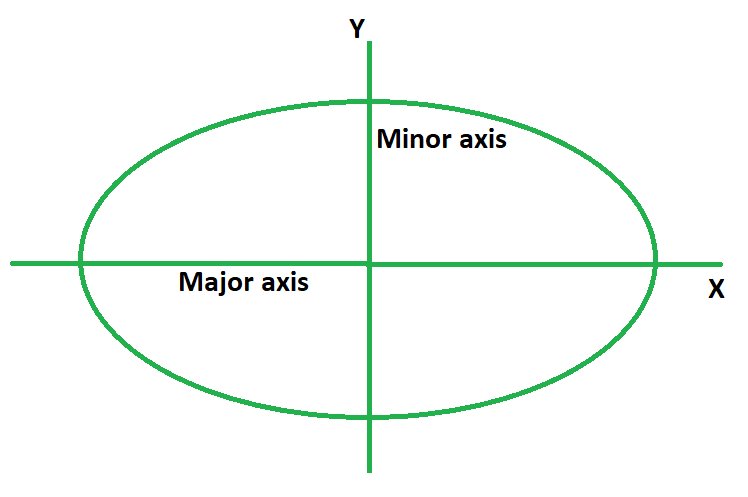# How to find the Perimeter and Area of Ellipse?

• Last Updated : 23 May, 2022

An ellipse is a two-dimensional curve on a plane that surrounds two focus points and is defined as the sum of the distances between the two focal points for every point on the curve. In other words, it’s a planar curve in which the total of the distances between its two focal points, or foci, is always the same from the given location. There are two sorts of axes in an ellipse, the major axis, and the minor axis. The main axis is the ellipse’s longest chord. The minor axis is orthogonal to the major axis and bisects the major axis in the middle.### How to find the Perimeter of an Ellipse?

The perimeter of an ellipse is defined as its boundary length. To put it another way, it is equal to the length of the continuous line forming the boundary of the given ellipse. It can also be interpreted as the circumference of the ellipse. Its formula is equal to 2π (a constant) times the square root of the value of squares of its semi-minor and semi-major axis divided by 2. It is denoted by the symbol P.

The formula to find out the perimeter of ellipse is given by,

P = 2π √((a2 + b2) / 2))

where,

• a is the length of semi-major axis,
• b is the length of semi-minor axis.

### How to find the Area of an Ellipse?

The area of an ellipse is defined as the amount of region occupied by it in a 2D space. It is denoted by the symbol A and its formula equals π (a constant) times the product of lengths of its semi-minor and semi-major axis.

The formula to calculate the area of an ellipse is:

A = πab

where,

• a is the length of semi-major axis,
• b is the length of semi-minor axis.

### Sample Problems

Problem 1: Calculate the perimeter of an ellipse with a semi-major axis of 12 cm and a semi-minor axis of 10 cm.

Solution:

We have,

a = 12

b = 10

Using the formula we have,

P = 2π √((a2 + b2) / 2))

= 2π √((122 + 102) / 2))

= 2π √(244/2)

= 2 × 3.14 × 11.04

= 69.33 cm

Problem 2: Calculate the perimeter of an ellipse with a semi-major axis of 10 cm and a semi-minor axis of 7 cm.

Solution:

We have,

a = 10

b = 7

Using the formula we have,

P = 2π √((a2 + b2) / 2))

= 2π √((102 + 72) / 2))

= 2π √(149/2)

= 2 × 3.14 × 8.63

= 54.20 cm

Problem 3: Calculate the perimeter of an ellipse with a major axis of 14 cm and a minor axis of 12 cm.

Solution:

We have,

a = 14/2 = 7

b = 12/2 = 6

Using the formula we have,

P = 2π √((a2 + b2) / 2))

= 2π √((72 + 62) / 2))

= 2π √(85/2)

= 2 × 3.14 × 6.51

= 40.94 cm

Problem 4: Calculate the perimeter of an ellipse with a major axis of 18 cm and a minor axis of 10 cm.

Solution:

We have,

a = 18/2 = 9

b = 10/2 = 5

Using the formula we have,

P = 2π √((a2 + b2) / 2))

= 2π √((92 + 52) / 2))

= 2π √(106/2)

= 2 × 3.14 × 7.28

= 45.71 cm

Problem 5: Calculate the area of an ellipse with a semi-major axis of 8 cm and a semi-minor axis of 3 cm.

Solution:

We have,

a = 8

b = 3

Using the formula we have,

A = πab

= 3.14 × 8 × 3

= 75.36 sq. cm

Problem 6: Calculate the area of an ellipse with a semi-major axis of 16 cm and a semi-minor axis of 11 cm.

Solution:

We have,

a = 16

b = 11

Using the formula we have,

A = πab

= 3.14 × 16 × 11

= 552.64 sq. cm

Problem 7: Calculate the area of an ellipse with a major axis of 10 cm and a minor axis of 8 cm.

Solution:

We have,

a = 10/2 = 5

b = 8/2 = 4

Using the formula we have,

A = πab

= 3.14 × 5 × 4

= 62.8 sq. cm

My Personal Notes arrow_drop_up
Recommended Articles
Page :